## Tamilnadu Samacheer Kalvi 10th Maths Solutions Chapter 2 Numbers and Sequences Ex 2.9

10th Maths Exercise 2.9 Samacheer Kalvi Question 1.
Find the sum of the following series
(i) 1 + 2 + 3 + … + 60
(ii) 3 + 6 + 9 + … + 96
(iii) 51 + 52 + 53 + … + 92
(iv) 1 + 4 + 9 + 16 + … + 225
(v) 62 + 72 + 82 + … + 212
(vi) 103 + 113 + 123 + … + 203
(vii) 1 + 3 + 5 + … + 71
Solution:
(i) 1 + 2 + 3 + ……… + 60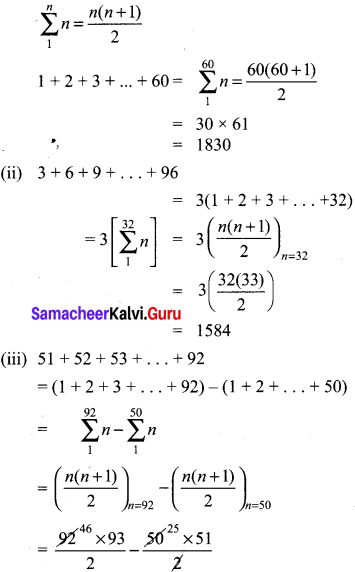= 4278 – 1275 = 3003
(iv) 1 + 4 + 9 + 16 + … + 225
= 12 + 22 + 32 + 42 + ……… + 152
$$\sum_{1}^{n} n^{2}=\frac{n(n+1)(2 n+1)}{6}$$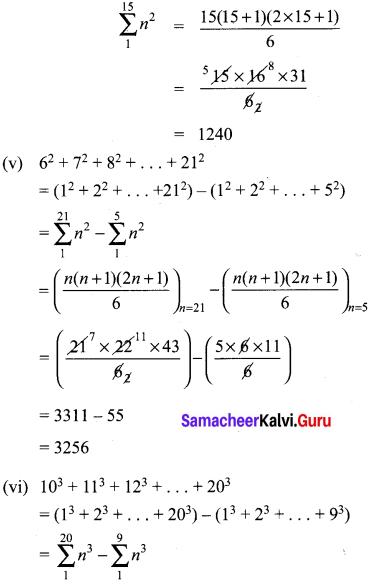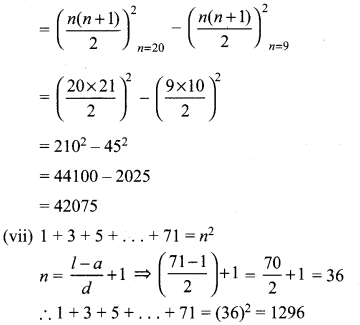Ex 2.9 Class 10 Samacheer Question 2.
If 1 + 2 + 3 + … + k = 325, then find 13 + 23 + 33 + …………. + K3.
Solution:
1 + 2 + 3 + … + K = 325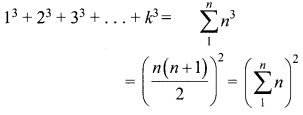If 1 + 2 + 3 … + k = 325
13 + 23 + 33 + … + K3 = (325)2 = 105625

10th Maths Exercise 2.9 Answers Question 3.
If 13 + 23 + 33 + … + K3 = 44100 then find 1 + 2 + 3 + … + k.
Solution:
If 13 + 23 + 33 + … + K3 = 44100
1 + 2 + 3 + … + K = $$\sqrt { 44100 }$$
= 210

Exercise 2.9 Class 10 Maths Question 4.
How many terms of the series 13 + 23 + 33 + … should be taken to get the sum 14400?
Solution:
13 + 23 + 33 + ……… + n3 = 14400
$$\left(\frac{n(n+1)}{2}\right)^{2}$$ = 14400 = (120)2
$$\frac{n(n+1)}{2}$$ = $$\sqrt { 14400 }$$ = 120
n(n + 1) = 240
Method 1:
n2 + n – 240 = 0
n2 + 16n – 15n – 240 = 0
n(n + 16) – 15(n + 16) = 0
(n + 16)(n – 15) = 0
n = -16, 15
∴ 15 terms to be taken to get the sum 14400.
Method 2:
n2 + n – 240 = 0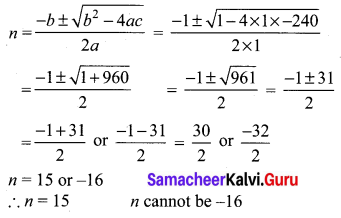Exercise 2.9 Class 10 Maths Samacheer Question 5.
The sum of the squares of the first n natural numbers is 285, while the sum of their cubes is 2025. Find the value of n.
Solution:
12 + 22 + 32 + …… + n2 = 285
13 + 23 + 33 + …… + n3 = 2025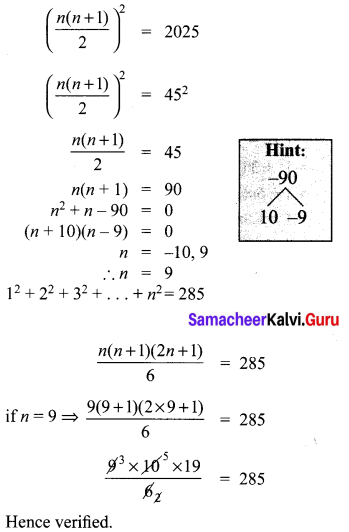10th Maths Exercise 2.9 Question 6.
Rekha has 15 square colour papers of sizes 10 cm, 11 cm, 12 cm,…, 24 cm. How much area can be decorated with these colour papers?
Solution:
102 + 112 + 122 + … + 242
= (12 + 22 + … + 242) – (12 + 22 + … + 92)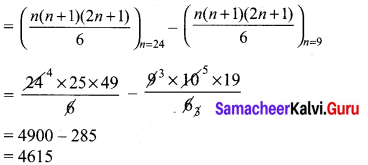∴ Rekha has 4615 cm2 colour papers. She can decorate 4615 cm2 area with these colour papers.

Ex 2.9 Class 10 Question 7.
Find the sum of the series (23 – 1) + (43 – 33) + (63 – 153) +… to (i) n terms (ii) 8 terms.
Solution:
(23 – 1) + (43 – 33) + (63 – 153) + ……… n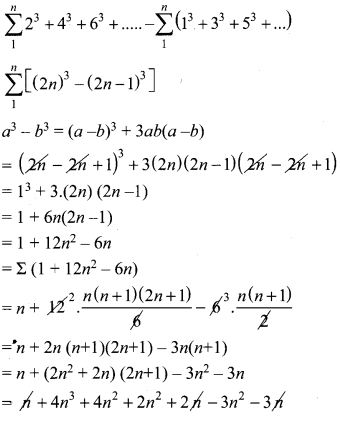= 4n3 + 3n2 = sum of ‘n’ terms.
When n = 8
Sum = 4 × 83 + 3 × 82
= 2048 + 192 = 2240